Finite Mathematics : Simple Interest

Example Questions

Example Question #1 : Simple Interest

What is the total interest made after 4 years on a simple interest loan that accumulates 13% each year and has original amount was $1350. Possible Answers: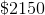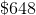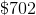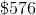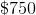Correct answer:Explanation: To solve this problem, first recall the formula to calculate simple interest.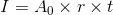where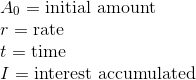From the question it's known that,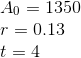Thus the equation becomes,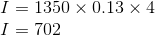Therefore$702 in interested accumulated after four years.